Highly Nonlinear, Dispersion Flattened Equiangular Spiral Photonic Crystal Fiber in Telecommunications Window | OMICS International
Journal of Lasers, Optics & Photonics

# Highly Nonlinear, Dispersion Flattened Equiangular Spiral Photonic Crystal Fiber in Telecommunications Window

Jamshid Heiadarialamdarloo1*, Carvalho MI2, Ricardo Oliviera2, Rogério Nunes Nogueira2 and Antonio Luis Jesus Teixeira2

1Instituto de Telecomunicações, Universidade de Aveiro, Campo Universitário, 3810-391 Aveiro, Portugal

2Faculty of Engineering Universidade do Porto (FEUP), Campus da FEUP, Rua Dr.Roberto Frias, 378, 4200-465 Porto, Portugal

*Corresponding Author:
Jamshid Heidarialamdarloo
Instituto de Telecomunicações, Universidade de Aveiro
Campo Universitário, 3810-391 Aveiro, Portugal
Tel: 962261298
E-mail: [email protected]

Received date: May 17, 2016; Accepted date: June 13, 2016; Published date: June 15, 2016

Citation: Heiadarialamdarloo J, Carvalho MI, Oliviera R, Nogueira RN, Teixeira ALJ (2016) Highly Nonlinear, Dispersion Flattened Equiangular Spiral Photonic Crystal Fiber in Telecommunications Window. J Laser Opt Photonics 3:131. doi:10.4172/2469-410X.1000131

Copyright: © 2016 Heiadarialamdarloo J, et al. This is an open-access article distributed under the terms of the Creative Commons Attribution License, which permits unrestricted use, distribution, and reproduction in any medium, provided the original author and source are credited.

Visit for more related articles at Journal of Lasers, Optics & Photonics

#### Abstract

This paper presents an equiangular spiral (ES) cladding photonic crystal fiber (PCF) made of lead silicate glass SF57. The proposed ES-PCF exhibits a flat dispersion at the telecommunications window with high nonlinearity and low confinement loss, showing an ultra-flattened dispersion at wavelengths ranging from 1.51 to 1.81 μm (300 nm band), with confinement losses of less than 0.0031 dB/km within this wavelength range, and two zero-dispersion wavelengths at 1.55 and 1.76 μm. Moreover, this fiber also has a high nonlinear coefficient of 1285 W-1km-1 at 1.55 μm.

#### Keywords

Photonic crystal fiber; High nonlinearity; Ultra-flattened dispersion; Confinement loss

#### Introduction

Photonic crystal fibers (PCFs) also known as microstructured or holey fibers were developed in early 1990s . In a PCF, the fiber core is surrounded by a microstructured cladding, typically consisting of air holes arranged according to a given geometry. The continuous and innovative theoretical and experimental works on the PCFs shows that they possess fundamentally exceptional properties and over comes many limitations of conventional optical fiber, i.e. being endlessly single mode, high nonlinear coefficients, dispersion engineering and guiding light through hollow core [1,2]. PCFs with a high effective nonlinear coefficient, flat dispersion and low loss are suitable for various applications in nonlinear optics, namely, super-continuum generation, all optical signal processing, and wavelength conversion . Light in PCFs is guided by the modified total internal reflection (MTIR) mechanism which is quite similar to the guiding mechanism in standard single mode fibers . Light is guided in a higher index core (generally pure silica), which results in light being confined into a small area. Due to the resulting high intensity of light into small mode area, the fiber can show a highly nonlinear behavior [3,4]. The nonlinear parameter can be further enhanced if the fiber is made using glasses with higher nonlinearities than pure silica, such as sulphur hexauoride, lead silicate, tellurite, bismuthes oxide, and chalcogenide glasses [5,6]. In particular, the lead silicate glasses (SF57) exhibit good thermal, crystallization stability and a high nonlinear refractive index, which makes them particularly attractive for microstructured fibers . A high nonlinearity can also be achieved with the smallest possible effective mode area, through an adequate choice of the materials and design of the fiber structure. The optical fiber dispersion profile is also of the utmost importance. The total dispersion is the sum of the material dispersion and the waveguide dispersion . The microstructured cladding of the index guiding fibers offers greatly enhanced design flexibility and can manipulate the dispersion characteristics by controlling structural parameters such as the air-hole diameter and the hole-to-hole spacing (pitch). Such dispersion engineering allows the PCF to be designed with a flat dispersion profile over the wide range of the wavelengths from visible region to telecommunication band. A large variety of fiber structures have also been proposed in recent years to flatten the dispersion profile and make it nearly zero at the telecommunications window. These include the square-holes structure PCF , the hexagonal structure PCF [8-10], and PCFs with circular  and octagonal designs . In all of the mentioned structures, the modification of some parameters, such as the separation between air holes (pitch) or the percentage of air, allows a fine tuning of their dispersion profile. Recently, a PCF with an equiangular spiral design made of SF57 glass was proposed by Arti Agrawal et al. . This model presents a very high nonlinear coefficient at 1064 nm and 1550 nm, namely, γ>5250 W-1km-1 and γ>2150 W-1km-1, respectively, and a low dispersion at 1060 nm, i.e., 0.795 ps/nm.km. Here, we study an ES-PCF made of SF57 with a structure consisting of holes with different diameter and including a first ring, which will be optimized to obtain flat dispersion at the telecommunications wavelength window. Our optimum design has a dispersion profile with two zeroes located at 1.55 μm and 1.76 μm, with an ultra-flattened dispersion at a wide range of wavelengths in the C and L telecom bands. It also exhibits a low confinement loss and a high nonlinear coefficient at the region of interest.

#### Mathematical Model

Figure 1 shows the proposed ES-PCF, which consists of a SF57 fiber with air holes in the cladding that are positioned in nine arms and five rings according to the ES equation that, in polar coordinates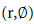, is r=bexp(φcot(α)) , where b is closely associated with radial distance and parameter α represents the constant angle that the radial direction at a given point of the ES curve makes with the tangent to that point. Another ring of smaller air holes is introduced closer to the fiber core, positioned at a distance r0 from the center. The angular separation between holes of the same ring and in consecutive spiral arms is θ=π/Narms. To avoid overlap between adjacent holes, the value of α should be carefully chosen. Here, we considered α=2(Narms–5) (Narms–2), which works well for our hole diameter and parameter b. In our study, we considered that the air holes in the outer rings of the ES have gradually increasing diameters (di), from d1=0.45 μm to d5=0.85 μm with a step of 0.10 μm. Furthermore, the position of these outer rings can be written as ri=k(i-1)r1, where i=2,…5, r1=1.1 μm, and k=1.227. On the other hand, the value of r0 and the diameter of the holes in the first ring will be optimized to obtain the desired optical properties for this PCF. The proposed structure also includes a very small air hole of radius r0, which is used to further tune the dispersion. The guided modes supported by this structure, as well as their effective refractive indexes, were obtained as a function of wavelength by solving the wave equation using commercial software .

Once the modes and the modal effective index are obtained, the dispersion and the nonlinear coefficient of this type of PCF can be easily determined. Effectively, from the transverse electric field Et(x,y), the nonlinear coefficient of the PCF can be calculated as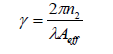(1)

Where n2 is the nonlinear refractive index and Aeff is the effective mode area that is given by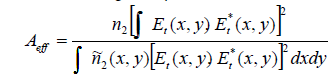(2)

In this expression,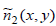is the nonlinear refractive index of the material positioned at (x,y). Note that the nonlinear refractive index for SF57 and for air is given by 4.1 × 10-19 (m2⁄W) and 3 × 10-23 (m2⁄W), respectively [13,16]. From the imaginary part of the effective refractive index, the confinement loss of the PCF in units of dB/m can be computed using the formula .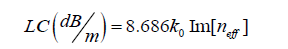(3)

Where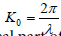is the free space wave number. On the other hand, from the real part of neff, we can evaluate the fiber dispersion as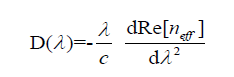(4)

Where c is the light velocity in vacuum. Our results have taken in account the material dispersion defined by the Sellmeier equation using the coefficients given for the commercially available lead silicate glass (Schott SF57) .

#### Simulations and Results

We started by studying the effect of adding an inner ring to a structure consisting of five rings. The air holes in the inner ring have diameter d0=0.3 μm and are positioned at a distance r0=0.75 μm from the center. An additional central hole with diameter rc=20 nm was also considered. We obtained the dispersion profile, the confinement loss, and the nonlinearity for each case and compared our results with those in reference  (Figure 2). As shown in Figure 2a, the dispersion profile of the proposed design with nine arms and five rings is flatter and closer to zero than the result presented in reference , obtained with seven arms and four rings, for the wavelengths at the telecommunications window region. Figure 2a also shows that the design with an inner ring exhibits a flatter dispersion. On the other hand, the confinement loss curves represented in Figure 2b clearly show that the loss with our design is significantly lower than that in reference . Furthermore, the structure with inner ring holes causes better field confinement. The confinement losses increase with the wavelength, implying, as expected, that the modes for longer wavelengths are less confined in the core. In turn, this also affects the nonlinear coefficient and causes its decrease with the wavelength, as can be observed in Figure 2c. The nonlinear coefficient for the structure with an inner ring is higher than the one for the structure without such ring. Our simulation results also showed that the nonlinearity remains approximately constant regardless of the number of rings used in the outer cladding. This suggests that the effective mode area of a structure with three or more outer rings is independent of the number of rings. The choice of ES-PCF structure, which has nine arms in five rings with unequal diameter for air holes and an inner ring, provides better control of dispersion curve, high nonlinearity, and lower confinement loss.

Figure 2: Dispersion (a) confinement loss (b) and nonlinear coefficient (c) for different structures.

The fact that the curves corresponding to the structures with and without the inner ring are quite different suggests that the careful adjustment of the geometric parameters of the inner ring will allow an optimization of the equiangular PCF properties. Therefore, in what follows, the influence of r0 and d0 in the optical properties of this PCF will be studied. Figure 3 depicts the dispersion curves for the proposed PCF with nine arms and five rings when different values of the inner ring radius and hole diameters are considered. Figure 3a shows the wavelength dependence of the dispersion for different values of the parameter r0 when d0=0.3 μm and rc=0.75 μm. The simulation results show that, under these conditions, as the r0 increases the dispersion increases and the zero dispersion shifts from 1.55 μm to lower wavelengths. For r0=0.735 μm, the dispersion curve attains values close to zero at a large wavelength band centered at 1.55 μm. We then proceed by considering the inner ring radius to have the optimum value r0=0.735 μm and investigate the effect of varying the air hole size in that ring. Figure 3b presents the dispersion profiles in this case, r0=0.735 μm and rc=0.075 μm, for various values of d0. From this figure, it can be observed that the increase of the air holes diameter in the inner ring is associated with the decrease of the dispersion at wavelengths around 1.55 μm. Furthermore, for the air hole diameter d0=0.31 μm, the dispersion curve is closer to zero at wavelengths in the telecommunication window around.

Figure 3: Dispersion curves of the ES-PCF (a) for different values of r0 when d0=0.30 µm and rc=0.075 µm (b) for different values of d0 when r0=0.735 µm and rc=0.075 µm.

As a final step in the optimization process, we took the inner ring radius and holes diameter to attain the optimum values mentioned above and computed the dispersion profiles for different central hole radius. Our results, depicted in Figure 4a, shows that increasing the central hole radius reduces the values of both the anomalous dispersion and dispersion slope near the zero dispersion wavelength. However, it also decreases the anomalous dispersion bandwidth, as can be observed in this figure. The best dispersion profile is obtained with the value of rc=0.0735 μm, which exhibits a dispersion profile with two zeros nearly at 1.55 and 1.76 μm, and ultra-flat dispersion at the wavelength range of 1.51 to 1.8 μm (300 band) with a maximum value of 2 ps/nm.km. Moreover, for these optimal parameters, values of the confinement loss below 0.0031 dB/km were observed in that wavelength region. We also obtained a nonlinear coefficient of 1285 W-1Km-1 at 1.55 μm. Figure 4b presents the electric field profile for the chosen parameters at 1.55 μm, and shows that in this case the mode is well confined within the area defined by the first ring.

Figure 4: : (a) Dispersion properties for the optimum result, (b) electric field profile of the fundamental mode at 1.55 µm.

#### Conclusions

In this work, a novel design of an ES-PCF has been proposed. The effect of varying the parameters of the PCF geometry, namely, the inner ring air hole diameter and distance from the center, and central hole diameter, in terms of the dispersion, nonlinearity and confinement loss has been investigated. The results show that lower values of dispersion and confinement loss can be obtained by considering the existence of an inner ring with smaller air holes. Our PCF model with lead silica can exhibit nearly zero dispersion at 1.55 μm and has ultra-flattened dispersion at wavelengths in the C and L telecommunication bands, while also possessing very low confinement loss and a high nonlinear coefficient. The dispersion and the confinement loss at the telecommunication window decreased significantly compared with that in reference .

#### Acknowledgements

This research was funded by FCT Portuguese government as a PhD scholarship under the contract number Pest-OE/EEI/LA0008/2013 with Instituto de Telecomunicações (IT) Aveiro.

#### References

Select your language of interest to view the total content in your interested language

### Article Usage

• Total views: 10285
• [From(publication date):
June-2016 - Feb 18, 2020]
• Breakdown by view type
• HTML page views : 10042Can't read the image? click here to refresh